xStatic spacetimeEncyclopedia
In general relativity
General relativity
General relativity or the general theory of relativity is the geometric theory of gravitation published by Albert Einstein in 1916. It is the current description of gravitation in modern physics...

, a spacetime
Spacetime
In physics, spacetime is any mathematical model that combines space and time into a single continuum. Spacetime is usually interpreted with space as being three-dimensional and time playing the role of a fourth dimension that is of a different sort from the spatial dimensions...

is said to be static if it admits a global, non-vanishing, timelike Killing vector field
Killing vector field
In mathematics, a Killing vector field , named after Wilhelm Killing, is a vector field on a Riemannian manifold that preserves the metric. Killing fields are the infinitesimal generators of isometries; that is, flows generated by Killing fields are continuous isometries of the manifold...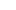which is irrotational, i.e., whose orthogonal distribution is involutive. (Note that the leaves of the associated foliation
Foliation
In mathematics, a foliation is a geometric device used to study manifolds, consisting of an integrable subbundle of the tangent bundle. A foliation looks locally like a decomposition of the manifold as a union of parallel submanifolds of smaller dimension....

are necessarily space-like hypersurface
Hypersurface
In geometry, a hypersurface is a generalization of the concept of hyperplane. Suppose an enveloping manifold M has n dimensions; then any submanifold of M of n − 1 dimensions is a hypersurface...

s.) Thus a static spacetime is a stationary spacetime
Stationary spacetime
In general relativity, specifically in the Einstein field equations, a spacetime is said to be stationary if it admits a Killing vector that is asymptotically timelike....

satisfying this additional integrability condition. These spacetimes form one of the simplest classes of Lorentzian manifolds.

Locally, every static spacetime looks like a standard static spacetime which is a Lorentzian warped product R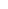S with a metric of the form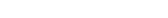,
where R is the real line,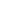is a (positive definite) metric and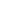is a positive function on the Riemannian manifold
Riemannian manifold
In Riemannian geometry and the differential geometry of surfaces, a Riemannian manifold or Riemannian space is a real differentiable manifold M in which each tangent space is equipped with an inner product g, a Riemannian metric, which varies smoothly from point to point...

S.

In such a local coordinate representation the Killing field
Killing vector field
In mathematics, a Killing vector field , named after Wilhelm Killing, is a vector field on a Riemannian manifold that preserves the metric. Killing fields are the infinitesimal generators of isometries; that is, flows generated by Killing fields are continuous isometries of the manifold...may be identified with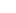and S, the manifold of-trajectories, may be regarded as the instantaneous 3-space of stationary observers. If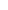is the square of the norm of the Killing vector field,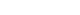, bothandare independent of time (in fact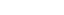). It is from the latter fact that a static spacetime obtains its name, as the geometry of the space-like slice S does not change over time.

## Examples of static spacetimes

1. The (exterior) Schwarzschild solution
2. de Sitter space
De Sitter space
In mathematics and physics, a de Sitter space is the analog in Minkowski space, or spacetime, of a sphere in ordinary, Euclidean space. The n-dimensional de Sitter space , denoted dS_n, is the Lorentzian manifold analog of an n-sphere ; it is maximally symmetric, has constant positive curvature,...

(the portion of it covered by the static patch).
3. Reissner-Nordström space
4. The Weyl solution, a static axisymmetric solution of the Einstein vacuum field equations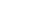discovered by Hermann Weyl
Hermann Weyl
Hermann Klaus Hugo Weyl was a German mathematician and theoretical physicist. Although much of his working life was spent in Zürich, Switzerland and then Princeton, he is associated with the University of Göttingen tradition of mathematics, represented by David Hilbert and Hermann Minkowski.His...